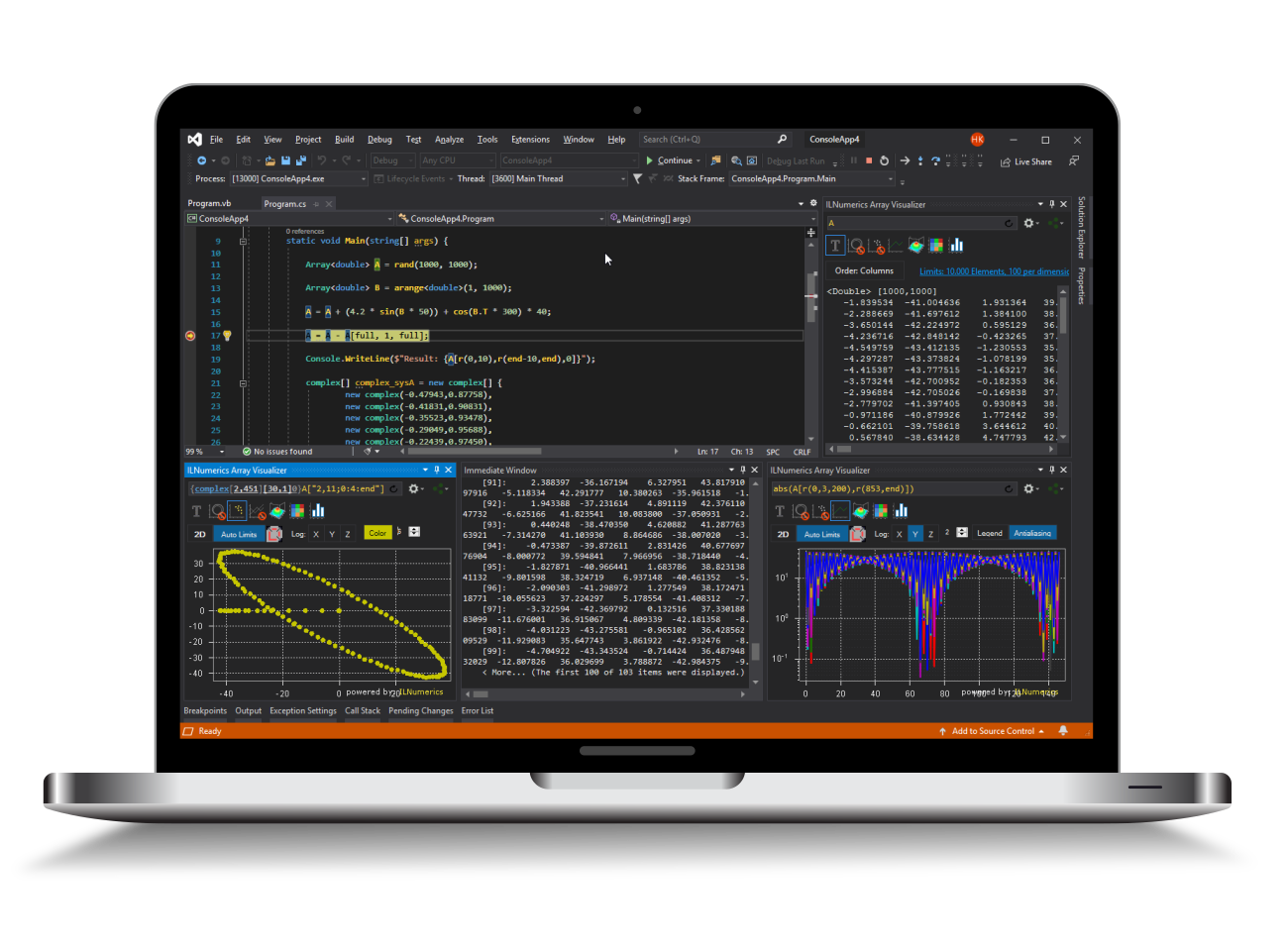Industrial Data Science
in C# and .NET:
Simple. Fast. Reliable.ILNumerics - Technical Computing

Modern High Performance Tools for Technical

Computing and Visualization in Industry and Science

# Controlling the Aspect Ratio

Properties of PlotCube allow to control the aspect ratio of displayed data dimensions and the size used to display the data. They allow to adopt the displayed result to multiple, partly conflicting requirements.

TL;DR

In order to make your 3D data look 'natural' and to maintain the original aspect ratio along the X-Y plane use:

• plotCube.ContentFitMode = ContentFitModes.ContentXY;
• plotCube.AspectRatioMode = AspectRatioMode.MaintainRatios;

In order to combine 'natural' data aspect ratio plots with rotation interaction in 3D make sure to configure your rendering area to be nearly square.

## Controlling the Space used for Rendering

In situations, where the available space for rendering on the form / the image is limited, the plot cube can be configured to use more space on the form for displaying the plots. The height or the width of the plots may be increased for better readablilty. This may lead to distortions of the generated plot. Now, for most 2D scenes / plots this distortion is acceptable or even desired.

Example:

In the above example, two plot cubes are generated. Both are configured to use one half of the available rendering space. The first plot cube uses the top area and the whole width. The second plot cube uses the bottom area and the same width. The thick green lines mark these areas (approximately). Their exact sizes are configured by PlotCube.ScreenRect, respectively.

The first plot cube uses StretchToFill as setting for its AspectRatioMode property. This leads the plot cube into trying to maximize the displayed output result. Note, how the plot cube's width uses up much of the horizontal space available within the upper ScreenRect. When determining the 'available space' PlotCube considers the actual size of the ScreenRect in both directions (screen X and screen Y). It does also take into account spaces required for rendering axis labels, ticks and tick labels as well as scale labels, where applicable.

In difference to that, the bottom plot was configured for AspectRatioMode = MaintainRatios. Here, the ratio of the space used for rendering in relation to the available space is kept the same in both directions: horizontally and vertically. What that means is: First, PlotCube determines the space available for rendering (again, taking all labels etc. into account) - for both directions individually. It then applies the smaller of the available space for rendering to both directions. Since there is less space available in vertical direction PlotCube uses the vertical space to properly size and render the plot cube's visual result. This setting is useful for interactive 3D scenes, where a user performs rotations of the plot cube. MaintainRatios makes sure that the plot cube remains its size (and aspect ratio), regardless which axis is currently aligned with horizontal or vertical spaces on the screen due to the current rotation setting:

Note, how the lower plot cube remains its overall shape while the upper plot cube undergoes heavy distortions during rotations.

MaintainRatios is the default value for plot cubes created for 3D mode. StretchToFill is the default value for plot cubes created for 2D mode (i.e: new PlotCube(twoDMode: true)).

## Content Aspect Ratio

In above examples only the aspect ratio of the horizontal and vertical spaces available for rendering have been considered. While those settings aid interactive rotations of the plot cube, they do not yet consider the actual aspect ratio of the data!

The term "plot cube" implies that a cube is used for hosting and labeling the data plots. The cube is the (virtual) 3D space limited by the main plot cube edge lines, the axes and grid lines and the various surrounding labels. The cube is fit into the area availabe for rendering, as determined by AspectRatioMode (see above). However, the data plots contained in the plot cube are fitted into a unit cube with aspect ratio 1:1:1 for its X / Y / Z dimensions, respectively. This way we ensure that all data are actually visible, regardless of their scale and position in the number space.

Especially for 3D plots, where at least two dimensions reflect real-world geometrical data it is often desired to reflect the natural aspect ratio within the visual result. For example, the height map of a slim, long, measured area ought to look like the original area, without distorting the XY plane to square size:

The property ContentFitMode determines how the data plots are fitted into the virtual 3D space called plot cube:

• UnitCube scales all dimensions of the data individually, in order to fit them exactly into the 1:1:1 unit cube. Naturally, this leads to distortion, if the natural data aspect ratio differs. But the rendering area will efficiently be filled.
• ContentXY applies general scaling as for ContentFitModes.UnitCube, but additionally shrinks one of the X or Y dimension of the plot cube so that the aspect ratio of the data in the X-Y plane is retained. Note, that the scaling in Z direction is not affected: data along the Z axis still fill the whole Z height of the plot cube (stretching or shrinking as required).
• ContentXYZ fits the data plot into the plot cube by retaining the data aspect ratio of all three axes. The longest axes will fit into the available rendering area for the plot cube. Both other axes will be shrunk accordingly, in order to maintain their data ratios, if required.
• Manual allows full control over the aspect ratio of the data plot as it is fit into the plot cube. The axes lengths of the data plot are scaled according to the setting of PlotCube.ContentFitRatios: a Vector3 property which is [1,1,1] by default. See below.

Example: manual aspect ratio configuration for a surface plot